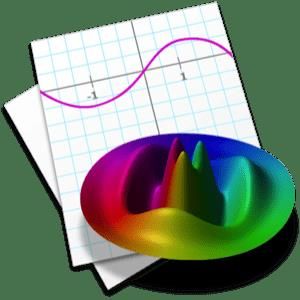# Graphing Calculator 5.3 macOS

#### mitsumi

##### Active MemberFile size: 22.79 MB

Graph equations in two and three dimensions.​

Graph explicit functions, implicit relations, parametric curves and surfaces, inequalities, points, vectors, contour plots, density plots, color maps, vector fields, ordinary differential equations, surfaces of revolution, coordinate transformations, conformal maps and complex-valued curves, surfaces, and ODEs.
Use rectangular, polar, cylindrical, or spherical coordinates. Solve equations numerically, graphically, or symbolically.
Symbolically evaluate indefinite and definite integrals, summations, products, and limits, simplify and expand trig identities, solve equations, systems of equations, inequalities, and differential equations symbolically, and factor polynomials.
Release Notes
Code:

Supported Operation System
• macOS 12.0 or later
• Apple Silicon or Intel Core processor

Homepage:
Code:

rapidgator.net:
Code: